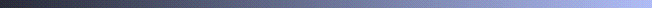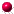## GSLIB Help Page: DECLUSDescription:
• Data are often spatially clustered; yet, we may need to have a histogram that is representative of the entire area of interest. To obtain a representative distribution, one approach is to assign declustering weights whereby values in areas/cells with more data receive less weight than those in sparsely sampled areas. The program declus provides an algorithm for determining 3-D declustering weights in cases where the clusters are known to be clustered preferentially in either high or low valued-areas. In other cases, polygon-type declustering weights might be considered whereby the weight is made proportional to the sample area (polygon) of influence.
• The declustering weights output by declusare standardized so that they sum to the number of data. A weight greater than 1.0 implies that the sample is being overweighted and a weight lesser than 1.0 implies that the sample is being downweighted (it is clustered with other samples).Parameters:
• datafl: a data file in simplified Geo-EAS format containing the variable to be declustered.
• icolx, icoly, icolz, and icolvr: the column numbers for the x y z coordinates and the variable.
• tmin and tmax: all values strictly less than tmin and strictly greater than tmax are ignored.
• sumfl: the output file containing all the attempted cell sizes and the associated declustered mean values.
• outfl: file for PostScript output.
• anisy and anisz: the anisotropy factors to consider rectangular cells. The cell size in the x direction is multiplied by these factors to get the cell size in the y and z directions, e.g., if a cell size of 10 is being considered and anisy2 and anisz3 then the cell size in the y direction is 20 and the cell size in the z direction is 30.
• minmax: an integer flag that specifies whether a minimum mean value (minmax=0) or maximum mean value (minmax=1) is being looked for.
• ncell, cmin, and cmax: the number of cell sizes to consider, the minimum size, and the maximum size. Note that these sizes apply directly to the x direction and the anis factors adjust the sizes in the y and z directions.
• noff: the number of origin offsets. Each of the ncell cell sizes are considered with noff different original starting points. This avoids erratic results caused by extreme values falling into specific cells. A good number is 4 in 2-D and 8 in 3-D. A short description of the program